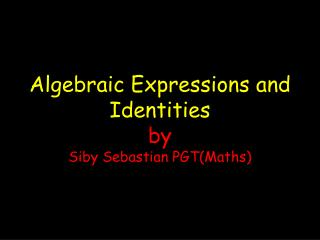# Algebraic Expressions and Identities by Siby Sebastian PGT(Maths) - PowerPoint PPT PresentationDownload PresentationAlgebraic Expressions and Identities by Siby Sebastian PGT(Maths)

Algebraic Expressions and Identities by Siby Sebastian PGT(Maths)Download Presentation## Algebraic Expressions and Identities by Siby Sebastian PGT(Maths)

- - - - - - - - - - - - - - - - - - - - - - - - - - - E N D - - - - - - - - - - - - - - - - - - - - - - - - - - -
##### Presentation Transcript

1. Mathematical Expressions We are familiar with the following type of numerical expressions: 12 + 6 3 x 12 6 (3 + 2) 15 - 4 (6)

2. What is a variable? Consider the expressions x + 12 3y – 2

3. What is a variable? x + 12 , 3y – 2 These expressions involve certain letters x and y . Any value can be assigned to these letters. A variable is a letter or symbol that represents an unknown value.

4. Algebraic Expressions When variables are used with other numbers, parentheses, or operations, they create an algebraic expression. x + 2 (a) (b) 3m + 6n - 6

5. What is a term? A term is the name given to a number, a variable, or a number and a variable combined by multiplication or division separated by the operation of addition or multiplication.

6. TERMS AlgebraicExpression a + 2 Terms a , 2 Algebraic Expression 3m + 6n - 6 Terms 3m, 6n, - 6

7. What are coefficients? A coefficient is the numerical factor of the term of an algebraic expression. Algebraic Expression Term Coefficient 6m + 5 6m 6 8r + 7m + 4 7m 7 14b - 8 14b 14

8. What are constants? A constant is a number in an expression that cannot change its value. In the expression: 5x + 7y - 2 the constant is - 2. Find the constant in 10ab - 4b+17a - 50 ?

9. Figure it out! Identify the terms, coefficients, and constants. 1. 12a - 6b + 4 2. 4x - 2y 3. c - 32 4. 3x + 2

10. Write algebraic expressions for these word phrases • Four more than s • The product of 7 and c • Nine less than x • A number divided by the sum of 4 and 7. • Twice the sum of a number plus 4. • The sum of ¾ of a number and 7. • Ten times a number increased by 150.

11. MONOMIALS 2x 3ab 4y2 These expressions have only one term. Such expressions are called MONOMIALS

12. BNOMIALS 2x -3y 3ab + 8b2 4y2 + 16z These are expressions having two terms. Such expressions are called BINOMIALS

13. TRINOMIALS 2x -3y -10 3ab + 8b2 + 20 4y2 + 16z -5 These are expressions having three terms. Such expressions are called TRINOMIALS

14. POLYNOMIALS Algebraic Expressions having one or more terms with non zero coefficients and non negative integral powers for the variable are called POLYNOMIALS 2x , 3x+15 , 2x-3y+2, 3xy – 4y + 2x3 +7y2 -10, 2x5 +4xy3 +10xy-3, 2ab + 4a2 – 6b2 - 2a - 3b + 2

15. LIKE TERMS Like terms are terms having same variables with same powers. But their coefficients may be different. Examples 1. 2xy , -5xy, 10xy, 2. 2a2b , -10 a2b, a2b

16. IDENTIFY LIKE TERMS 3x3yz , 4x, -5y , x3 , 5xy, -5xyx3, 2y , -7xyz, 10x, 27xy, 22y , 6yz

17. OPERATIONS ON POLYNOMIALS POLYNOMIALS CAN BE 1.ADDED 2.SUBTRACTED 3.MULTIPLIED AND 4.DIVIDED

18. QUIZ • In the expression 3x+y2 name the terms. 2. What is the coefficient of -3xy? • Are 2xy and 2x2y like terms? • Are 3x2y and -2yx2 like terms?

19. QUIZ 5. What is the maximum and minimum number of terms in a polynomial? 6. Is 5 an algebraic expression? 7. What are the variables in the equation 7x2y + 8xy2 ? Are they like terms?